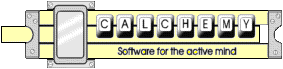# Solve By Dimensional Analysis or... What's With the Semicolons?

In the following example the semicolon operator is used in place of the "more conventional" arithmetic operators * or /. The ; informs Calchemy that it should determine the correct operation by performing a dimensional analysis of the equation. We call this Solve By Dimensional Analysis. In essence you just enter the parameters you know effect the result, separated by semicolons, and Calchemy derives the equation for you.

Water heater recovery time:
```40 gal;  1 cal/cc deltaC;  (140-45) deltaF;  40000 Btu/hour ? min
= 47.5686 min
```
The first line is what you type. The second line is the solution. What you type are the quantities that effect the result your looking for, a "?", and the units you want the result expressed in. In many cases, as with this problem, Calchemy is able to derive the mathematical equation relating the variables for you, by dimensional analysis. That is, Calchemy uses the Units of the quantities, and their Physical relationship to obtain the solution.

Rather remarkable isn't it?

Naturally you could have entered the exact conversion yourself like this:

```(40 gal * (1 cal/cc deltaC) * (140-45) deltaF) / (40000 Btu/hour) ? min
= 47.5686 min
```
But that's harder, and you have to parenthesize more.

If you would like to see the equation that Calchemy actually solved, you can mark Show Interpretations on the Calchemy Live equation entry form. For the water heater problem Calchemy responds with:

```40 gal;  1 cal/cc deltaC;  (140-45) deltaF;  40000 Btu/hour ? min
([{40 * gallon} * {(1 * calorie) / (cc * deltaC)}] * [{140 - 45} * deltaF]) * ([40000 * btu] / hour)^-1 ? minute
= 47.5686 min
```
Of course there are some limitations, so you must be careful, and if it's important CHECK YOUR RESULTS, but if you understand how it works you can save yourself a lot of time and trouble. Confusion crops up when the units of two parameters, separated by semicolons, cancel. In this case there will be multiple solutions, and you have to know your problem.

Calchemy Software Inc.
Fort Collins, COLast Updated 6/21/2009